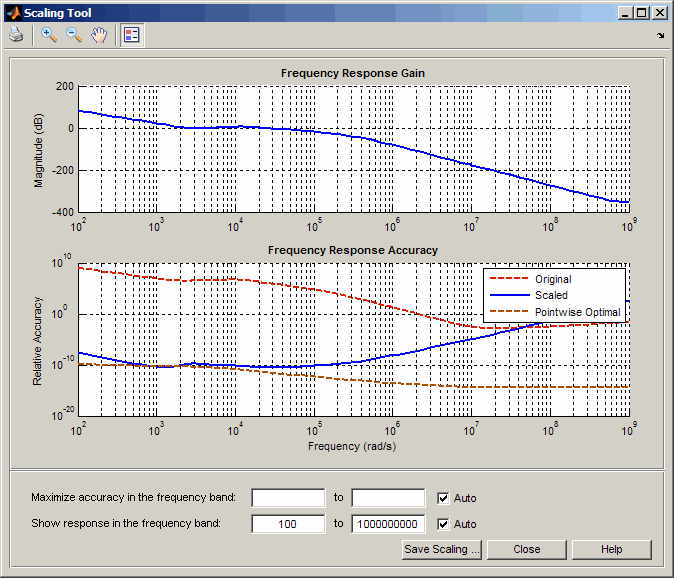prescale

Optimal scaling of state-space models

Syntax

scaledsys = prescale(sys)
scaledsys = prescale(sys,focus)
[scaledsys,info] = prescale(...)
prescale(sys)

Description

scaledsys = prescale(sys) scales the entries of the state vector of a state-space model sys to maximize the accuracy of subsequent frequency-domain analysis. The scaled model scaledsys is equivalent to sys.

scaledsys = prescale(sys,focus) specifies a frequency interval focus = {fmin,fmax} (in rad/TimeUnit, where TimeUnit is the system's time units specified in the TimeUnit property of sys) over which to maximize accuracy. This is useful when sys has a combination of slow and fast dynamics and scaling cannot achieve high accuracy over the entire dynamic range. By default, prescale attempts to maximize accuracy in the frequency band with dominant dynamics.

[scaledsys,info] = prescale(...) also returns a structure info with the fields shown in the following table.

 SL Left scaling factors SR Right scaling factors Freqs Frequencies used to test accuracy RelAcc Guaranteed relative accuracy at these frequencies

The test frequencies lie in the frequency interval focus when specified. The scaled state-space matrices are

$\begin{array}{l}{A}_{s}={T}_{L}A{T}_{R}\\ {B}_{s}={T}_{L}B\\ {C}_{s}=C{T}_{R}\\ {E}_{s}={T}_{L}E{T}_{R}\end{array}$

where TL = diag(SL) and TR = diag(SR). TL and TR are inverse of each other for explicit models (E = [ ]).

prescale(sys) opens an interactive GUI for:

• Visualizing accuracy trade-offs for sys.

• Adjusting the frequency interval where the accuracy of sys is maximized.For more information on scaling and using the Scaling Tool GUI, see Scaling State-Space Models.

Tips

Most frequency-domain analysis commands perform automatic scaling equivalent to scaledsys = prescale(sys).

You do not need to scale for time-domain simulations and doing so may invalidate the initial condition x0 used in initial and lsim simulations.